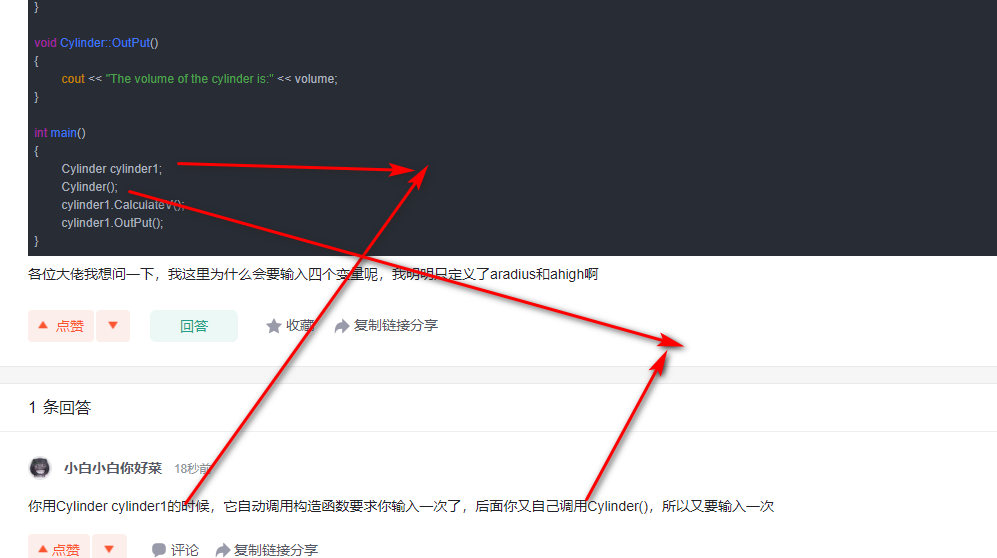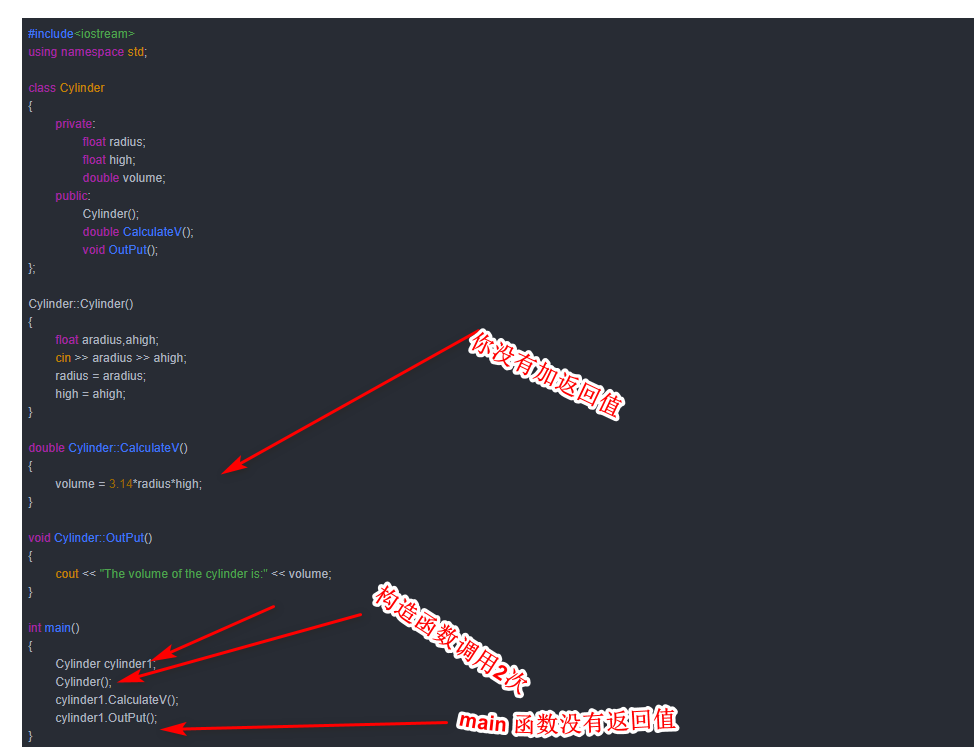2021-03-07 12:09

# 萌新C++求大佬们解惑（舅舅孩子）

``````#include<iostream>
using namespace std;

class Cylinder
{
private:
float radius;
float high;
double volume;
public:
Cylinder();
double CalculateV();
void OutPut();
};

Cylinder::Cylinder()
{
float aradius,ahigh;
cin >> aradius >> ahigh;
radius = aradius;
high = ahigh;
}

double Cylinder::CalculateV()
{
volume = 3.14*radius*high;
}

void Cylinder::OutPut()
{
cout << "The volume of the cylinder is:" << volume;
}

int main()
{
Cylinder cylinder1;
Cylinder();
cylinder1.CalculateV();
cylinder1.OutPut();
}``````

• 点赞
• 写回答
• 关注问题
• 收藏
• 复制链接分享
• 邀请回答

#### 4条回答

• 😭

点赞 评论 复制链接分享
• 谢谢大佬们，菜鸡明白了👍

点赞 评论 复制链接分享
•点赞 评论 复制链接分享
• 你用Cylinder cylinder1的时候，它自动调用构造函数要求你输入一次了，后面你又自己调用Cylinder()，所以又要输入一次

你后面单独的调用Cylinder，对cylinder.radius和cylinder.high的值不影响

点赞 评论 复制链接分享